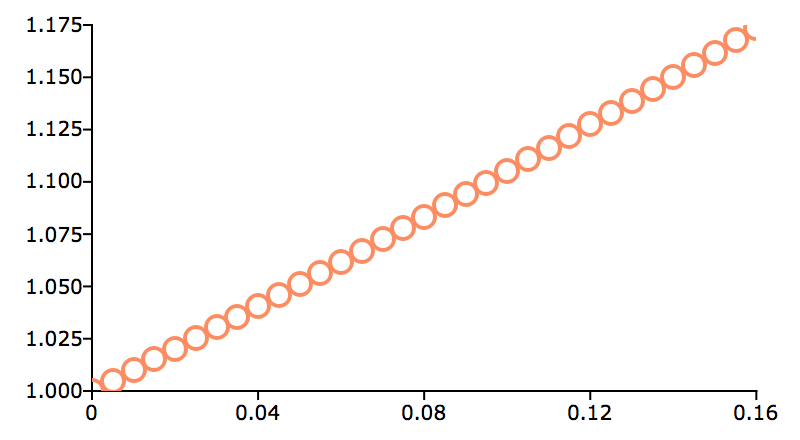# plotSetYticLabel¶

## Purpose¶

Controls the formatting and angle of Y-axis tick labels for 2-D graphs.

## Format¶

plotSetYticLabel(&myPlot, fmt[, angle])
Parameters: &myPlot (struct pointer) – A plotControl structure pointer. fmt (string or 2x1 string array) – the desired formatting for the Y-axis tick labels. If a 2x1 string array is passed in, the first element of fmt will control the left Y-axis and the second element will control the right Y-axis. sprintf() style formatting strings are supported. See Remarks below for more information. angle (Scalar) – Optional argument, the angle in degrees at which to display the Y-axis tick labels.

## Examples¶

### Basic usage¶

// Declare and initialize plotControl structure
struct plotControl myPlot;
myPlot = plotGetDefaults("scatter");

x = seqa(0, 0.005, 33);
y = exp(x);

// Set the Y-tick format to be decimal
// with three places after the decimal point,
// i.e. 1.050, 1.010, etc, for all tick labels
plotSetYticLabel(&myPlot, "%.3f");

// Draw the scatter plot
plotScatter(myPlot, x, y);


The code above produces a graph with Y-tick labels like the image below:Y-tick labels

Changing to format string to "%g" will change the labels to appear like this:

1.175
1.15
1.125
1.1
1.075
1.05
1.025


Changing to format string to "%.3e" will change the labels to appear like this:

1.175e+00
1.150e+00
1.125e+00
1.100e+00
1.075e+00
1.050e+00
1.025e+00


### Left and right Y-axis formatting.¶

// Create 2 vectors to plot
temperature = { 14,
31,
27,
19,
22 };

pct = { 6.3,
10.4,
13.2,
80.7,
28.5 };

X = seqa(1, 1, 5);

// Declare plotControl structure and fill
// with default values for 'xy' plots
struct plotControl myPlot;
myPlot = plotGetDefaults("xy");

// Use the left Y-axis for the first line
// and the right Y-axis for the second line
plotSetWhichYAxis(&myPlot, "left" $| "right"); /* ** Set the format for the tick labels ** of each Y-axis ** ** "%d°" - Print the numbers as integers, followed by ** the degree symbol (° is HTML). ** ** "%.1f%%" - Print one number after the decimal, followed by ** a percent sign. */ fmt = "%d°"$| /* format for left Y-axis */
"%.1f%%";     /* format for right Y-axis */

plotSetYTicLabel(&myPlot, fmt);

// Draw the plot using the settings applied above
plotXY(myPlot, X, temperature ~ pct);


This will give axes labels which look similar to below:

Left Y-axis 32°    Right Y-axis 90.0%
28°                 80.0%


## Remarks¶

The parameters of a sprintf() style format string are:

• precision - a literal scalar numeral, the number of digits after the decimal point

• format type - one of the following letters:

 d integer e scientific notation f floating point/decimal g either scientific or decimal, whichever is most compact

The format of the format string is:

"%.<precision><format type>"


Below are some examples of different format strings and how they would represent $$\pi$$

 “%.2f” 3.14 “%.4f” 3.1415 “%.2e” 3.14 “%.2g” 3.14 “%d” 3

This function sets an attribute in a plotControl structure. It does not affect an existing graph, or a new graph drawn using the default settings that are accessible from the Tools > Preferences > Graphics menu. See the GAUSS Graphics chapter for more information on the methods available for customizing your graphs.